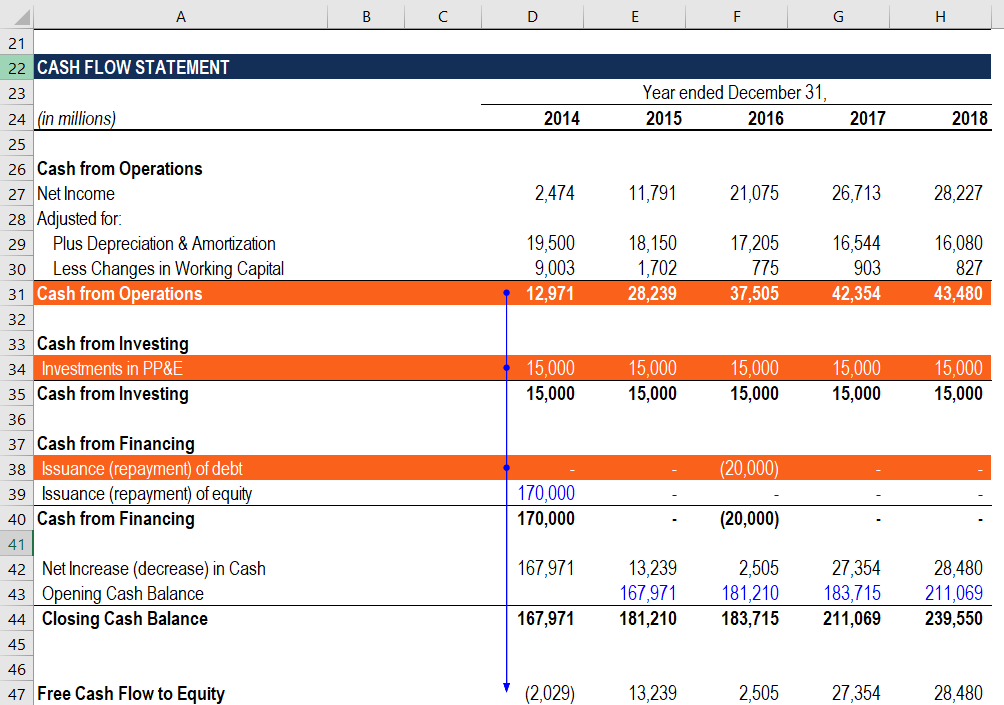# How to Calculate FCFE from CFO?

FCFE = CFO – CapEx + Net Borrowing

## Free Cash Flow to Equity (FCFE) from CFO?

Free Cash Flow to Equity (FCFE) is the amount of cash generated by a company that can be potentially distributed to its shareholders – you can calculate FCFE from CFO (cash flow from operations). FCFE is a key metric in one of the approaches in the Discounted Cash Flow (DCF) valuation model. Using the FCFE, an analyst can determine the Net Present Value (NPV) of a company’s equity, which can be subsequently used to calculate the theoretical share price of the company.

FCFE is different from Free Cash Flow to Firm (FCFF), which indicates the amount of cash generated to all holders of the company’s securities (both investors and lenders).### FCFE from CFO Formula

One of the approaches to calculating free cash flow to equity is based on the use of cash flow from operations (CFO) from the company’s cash flow statement. Cash flow from operations (also known as operating cash flow) is the amount of cash generated by a company from its ongoing business activities, excluding any financing and investing activities.

Essentially, the abovementioned approach is a shortcut to the FCFE from net income approach. Recall that the cash from operations is calculated using the formula below:

##### CFO = Net Income + Depreciation & Amortization – ΔWorking Capital

Where:

• CFO – Cash flow from operations
• ΔWorking Capital – Change in working capital

At the same time, FCFE from net income is calculated through the following formula:

##### FCFE = Net Income + Depreciation & Amortization – ΔWorking Capital – CapEx + Net Borrowing

Thus, we can rewrite the formula above by substituting the first three variables with the cash flow from operations (CFO) figure:

##### FCFE = CFO – CapEx + Net Borrowing

The formula above provides a simpler approach for calculating FCFE as it reduces the number of variables employed. Due to this reason, the calculation method is more suitable in a financial model as it makes the model more coherent and comprehensible by simplifying the calculations within a model.

### FCFE from CFO Formula and Financial Statements

An analyst who calculates the free cash flows to equity in a financial model must be able to quickly navigate through a company’s financial statements. The primary reason is that all inputs required for the calculation of the metric are taken from the financial statements. The guidance below will help you to quickly and correctly incorporate the FCFE from CFO calculation into a financial model.

1. Cash flow from operations (CFO): The CFO metric is calculated in the first section of the company’s cash flow statement.
2. CapEx: The capital expenditure (CapEx) can be found on the cash flow statement within the Cash from Investing section.
3. Net borrowing: Net debt amount is also located on the cash flow statement under Cash from Financing section.

Thank you for reading CFI’s guide to how to calculate FCFE from CFO. CFI offers the Financial Modeling & Valuation Analyst (FMVA)™ certification program for those looking to take their careers to the next level. To keep learning and advancing your career, the following CFI resources will be helpful:

• Capital Expenditure
• Financial Modeling Best Practices
• Stockholders Equity
• Three Financial Statements